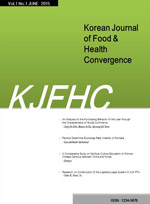상세검색
최근 검색어 전체 삭제
다국어입력
즐겨찾기0KCI등재후보 학술저널

# Proposal of VO2max estimation formula for elderly men and women using functional performance measurement

• 등재여부 : KCI등재후보
• 2022.02
• 21 - 30 (10 pages)
• 2

This study proposed a multiple regression equation for predicting VO2max of elderly men and women using functional performance variables required to conduct daily activities. The subjects of this study were 58 elderly men (72.4±5.9 yrs) and 117 elderly women (73.4±4.5 yrs) aged 65-90 who belong to the senior welfare center. The maximal graded exercise test using a cycle ergometer and functional performance representing muscle strength, endurance, static and dynamic flexibility, mobility, and agility were measured. For statistical processing, multiple regression analysis was performed, and the statistical significance level was α = .05. As a result, the VO2max estimation formula for the elderly was 0.419 (standing up and sitting down a chair) + 0.199 (leg endurance against wall) + 5.383, and R2=0.406. In addition, the VO2max estimation formula for elderly women is - 0.737 (standing up from a supine position) - 0.144 (waking around two cones in a figure 8) - 0.135 (%body fat) + 0.042 (one leg balance with eyes open) + 29.395, R2=0.367 was calculated. The conclusion is that if the maximal graded exercise test is not available, it is considered that VO2max of the elderly can be predicted properly by using the estimation formula calculated based on the functional performance variable.

1. Introduction

2. Research method

3. Result

4. Discussion

5. Conclusion

로딩중